### Home > CALC > Chapter Ch12 > Lesson 12.3.1 > Problem12-108

12-108.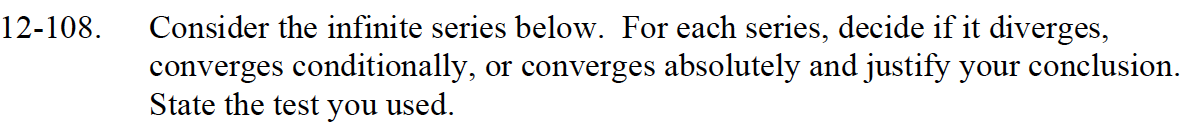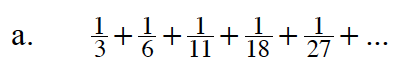$=\sum_{n=1}^\infty \frac{1}{n^2+2}$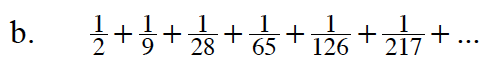$=\sum_{n=1}^\infty \frac{1}{n^3+1}$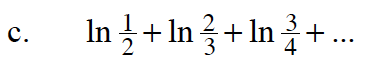$= \ln(1)-\ln(2)+\ln(2)-\ln(3)+\ln(3)-\ln(4)+...$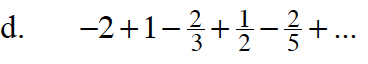Think of this as two separate series:

$\frac{-2}{1}+\frac{-2}{3}+\frac{-2}{5}+...\frac{1}{1}+\frac{1}{2}+\frac{1}{3}+...$